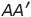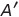Select Page

# Relations and Functions CBSE Maths 12 Science MCQ Answers in English

Relations and Functions CBSE Maths 12 Science MCQ Answers in English to enable students to get Answers in a narrative video format for the specific question.

Expert Teacher provides Relations and Functions CBSE Maths 12 Science MCQ Answers through Video Answers in English language. This video solution will be useful for students to understand how to write an answer in exam in order to score more marks. This teacher uses a narrative style for a question from Relations and Functions not only to explain the proper method of answering question, but deriving right answer too.

Please find the question below and view the Answer in a narrative video format.

Question:

## Similar Questions from CBSE, 12th Science, Maths, Relations and Functions

Question 1 : Ifis a relation on N, write the range of R. (View Answer Video)

Question 2 : If f and g two functions such that they are one-one then g o f is: (View Answer Video)

Question 3 : Let A = {1, 2, 3}. Then, number of relations containing (1, 2) and (1, 3) which are reflexive and symmetric but not transitive is, (View Answer Video)

Question 4 : Letdefined as f(x) = x be an identity function. Then, (View Answer Video)

### Determinant

Question 2 : Find the values of x, if. (View Answer Video)

Question 3 : Let, where. THhen (View Answer Video)

Question 4 : Find the inverse of the matrix. (View Answer Video)

Question 5 : Let A be square matrix of order 3 * 3, then |kA|is equal to (View Answer Video)

### Differential Equations

Question 1 :  Find the particular solution of the differential equationgiven thatWhen x =1.  (View Answer Video)

Question 2 : Find the general solution of differential equation(View Answer Video)

Question 3 : Write the sum of the order and degree of the differential equation(View Answer Video)

Question 4 : Write the differential equation formed from the equation y = mx + c, where m and c are arbitrary constants.

Question 5 : Solve the differential equation:(View Answer Video)

### Matrices

Question 1 : If, write, whereis the transpose of A? (View Answer Video)

Question 2 : Find the value of c from the equation:(View Answer Video)

Question 3 : Find the value of y, from the equation:(View Answer Video)

Question 4 : Find the value of z from the equation:(View Answer Video)

Question 5 : If, write the value of"x". (View Answer Video)